## Example Questions

← Previous 1 3 4

### Example Question #1 : Algebraic Functions

If f(x)=3x and g(x)=2x+2, what is the value of f(g(x)) when x=3?

20

22

24

18

24

Explanation:

With composition of functions (as with the order of operations) we perform what is inside of the parentheses first. So, g(3)=2(3)+2=8 and then f(8)=24.

### Example Question #1 : How To Find F(X)

g(x) = 4x – 3

h(x) = .25πx + 5

If f(x)=g(h(x)). What is f(1)?

4

19π – 3

42

π + 17

13π + 3

π + 17

Explanation:

First, input the function of h into g. So f(x) = 4(.25πx + 5) – 3, then simplify this expression f(x) = πx + 20 – 3 (leave in terms of π since our answers are in terms of π). Then plug in 1 for x to get π + 17.

### Example Question #1 : How To Find F(X)

If 7y = 4x - 12, then x =

(7y+12)/4
(7y-12)/4
(7y+3)/12
(7y+12)/3
Explanation:

Adding 12 to both sides and dividing by 4 yields (7y+12)/4.

### Example Question #1 : How To Find F(X)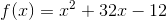What is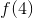?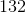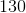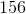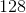Explanation: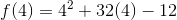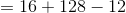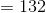### Example Question #2 : How To Find F(X)

If F(x) = 2x2 + 3 and G(x) = x – 3, what is F(G(x))?

6x2 + 5x

2x2 – 12x +21

2x2

6x2 – 12x

2x2 + 12x +18

2x2 – 12x +21

Explanation:

A composite function substitutes one function into another function and then simplifies the resulting expression.  F(G(x)) means the G(x) gets put into F(x).

F(G(x)) = 2(x – 3)2 + 3 = 2(x2 – 6x +9) + 3 = 2x2 – 12x + 18 + 3 = 2x2 – 12x + 21

G(F(x)) = (2x2 +3) – 3 = 2x2

### Example Question #1 : How To Find F(X)

If a(x) = 2x+ x, and b(x) = –2x, what is a(b(2))?

–503

132

503

128

–132

–132

Explanation:

When functions are set up within other functions like in this problem, the function closest to the given variable is performed first. The value obtained from this function is then plugged in as the variable in the outside function. Since b(x) = –2x, and x = 2, the value we obtain from b(x) is –4. We then plug this value in for x in the a(x) function. So a(x) then becomes 2(–43) + (–4), which equals –132.

### Example Question #1 : How To Find F(X)

Let F(x) = x3 + 2x2 – 3 and G(x) = x + 5.  Find F(G(x))

x3 + 2x2 – x – 8

x3x2x + 8

x3 + x2 + 2

x3 + 17x2 + 95x + 172

x3 + 2x2 + x + 2

x3 + 17x2 + 95x + 172

Explanation:

F(G(x)) is a composite function where the expression G(x) is substituted in for x in F(x)

F(G(x)) = (x + 5)3 + 2(x + 5)2 – 3 = x3 + 17x2 + 95x + 172

G(F(x)) = x3 + x2 + 2

F(x) – G(x) = x3 + 2x2 – x – 8

F(x) + G(x) =  x3 + 2x2 + x + 2

### Example Question #1 : How To Find F(X)

What is the value of xy2(xy – 3xy) given that = –3 and = 7?

–2881

–6174

2881

3565

–6174

Explanation:

Evaluating yields –6174.

–147(–21 + 63) =

–147 * 42 = –6174

### Example Question #2 : How To Find F(X)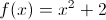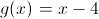Find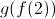.Explanation:is. To start, we find that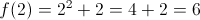. Using this, we find that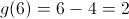.

Alternatively, we can find that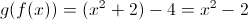. Then, we find that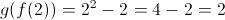.

### Example Question #1 : How To Find F(X)

It takes no more than 40 minutes to run a race, but at least 30 minutes. What equation will model this in m minutes?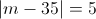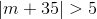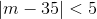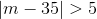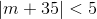If we take the mean number of minutes to be 35, then we need an equation which is less than 5 from either side of 35. If we subtract 35 from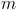minutes and take the absolute value, this will give us our equation since we know that the time it takes to run the marathon is between 30 and 40 minutes.